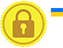# CSS grid-template

CSS grid-template property is a shorthand property, used to set the grids areas, rows and columns.

But using CSS grid , sub properties of an grid cannot be declared.

grid-template shorthand properties are

• grid-template-rows : It is used to set the size of the rows.
• grid-template-columns : It is used to set the size of the columns.
• grid-template-areas : It is used to set specified grid areas.

### Example

Try Yourself

For each grid, there are number of grid areas, to create it we use CSS grid-area property.

In the above example, for each div we assign grid names ( a1, a2, a3, a4, a5 ) using grid-area.

Then in #main_div we have created a template using created grid-area names.

In the grid-template: property we declared,

```". a1 ." 50px
"a2 a3 a4" 75px
"a5 a5 a5" 50px;```

Line 1: ". a1 ." 50px

• . - It denotes that the first cell must be an empty cell in the grid.
• a1 - It denotes an id value of div1 (because grid-area: a1 is decalred under div1).
• . - It denotes that the third cell must be an empty cell in the grid.
• 50px - It denotes the height of the first row.

Line 2: "a2 a3 a4" 75px

• a2 - It denotes an id value of div2 (because grid-area: a2 is decalred under div2).
• a3 - It denotes an id value of div3 (because grid-area: a3 is decalred under div3).
• a4 - It denotes an id value of div4 (because grid-area: a4 is decalred under div4).
• 75px - It denotes the height of the first row.

Line 3: "a5 a5 a5" 50px

• a5 - It denotes an id value of div5 (because grid-area: a5 is decalred under div5).
• 75px - It denotes the height of the first row.
• a5 is declared in three cells, so the whole row will be occupied by the a5 content.